# Semiconductor Laser Physics

Under normal conditions, all materials absorb light rather than emit it. The absorption process can be understood by referring the figure (a) below, where the energy levels E1 and E2 correspond to the ground state and the excited state of atoms of the absorbing medium. If the photon energy hν of the incident light of frequency ν is about the same as the energy difference Eg E2 - E1, the photon is absorbed by the atom, which ends up in the excited state. Incident light is attenuated as a result of many such absorption events occurring inside the medium.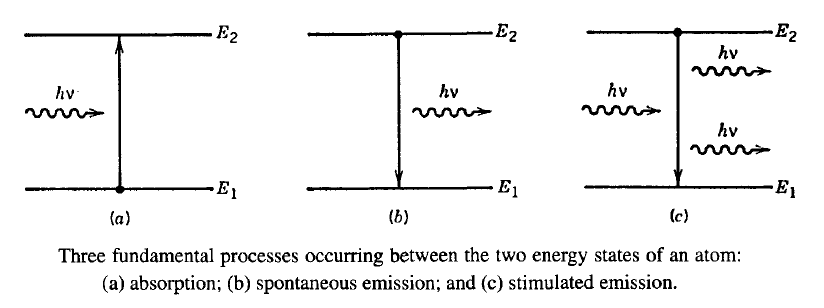#### 1. Spontaneous and Stimulated Emissions

If an atom is in the excited state, it eventually returns to its normal "ground" state and emits light in the process. Light emission can occur through two fundamental processes known as "spontaneous emission" and "stimulated emission". Both are shown schematically in the figure above. In the case of spontaneous emission, photons are emitted in random directions with no phase relationship among them. Stimulated emission, by contrast, is initiated by an existing photon. The remarkable feature of stimulated emission is that the emitted photon matches the original photon not only in energy (or in frequency), but also in its other characteristics, such as the direction of propagation. All lasers, including semiconductor lasers, emit light through the process of stimulated emission and are said to emit coherent light. In contrast, LEDs emit light through the incoherent process of spontaneous emission.

In a semiconductor laser, atoms participating in the process of stimulated emission are arranged in a crystal lattice. As a result, individual energy levels associated with a single atom merge and form energy bands. The two bands relevant for our discussion are the valence and conduction bands representing, respectively, the topmost band filled with electrons and the first band that is nearly empty of electrons. It is common to represent these bands in a E-k diagram shown schematically in the figure below, where k = p/ħ is the wave number of an electron with momentum p and energy E.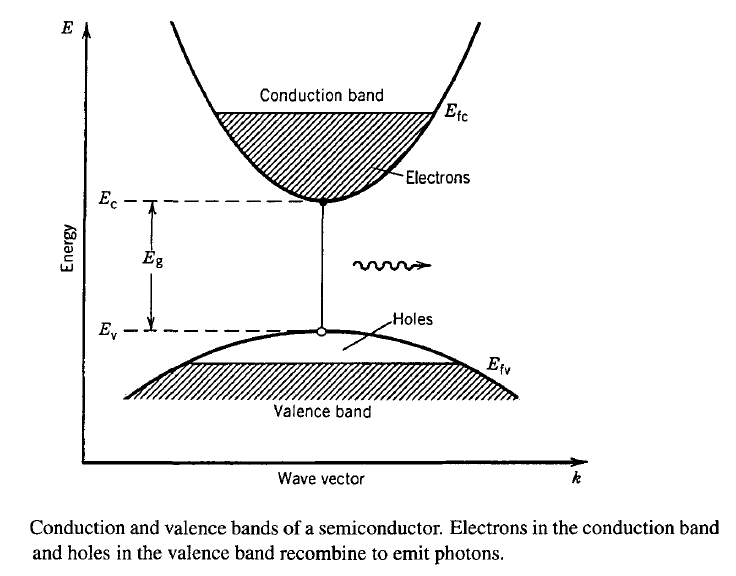If some of the electrons are raised to the conduction band through electrical pumping, the empty states left behind in the valence band represent holes. Light is produced when an electron near the bottom of the conduction band recombines with a hole in the valence band. The photon emitted during this recombination process carries an energy  ≈ Eg, where Eg is the bandgap energy of the semiconductor. Using ν = c/λ, one can conclude that a semiconductor laser can operate only in a certain wavelength region near λ = hc/Eg. For a semiconductor laser to emit light near 1.55 μm, its bandgap must be about 0.8 eV wide.

Semiconductor lasers operating in the wavelength range 1.3-1.6 μm were developed during the 1980s and are used almost exclusively for fiber-optic communications. They make use of a quaternary compound In1-xGaxAsyP1-y that is grown in a layer form on InP substrates using a suitable epitaxial growth technique. The lattice constant of each layer should remain matched to that of InP to maintain a well-defined lattice structure so that defects are not formed at the interfaces between any two layers with different bandgaps. The fraction x and y cannot be chosen arbitrarily but are related as x/y = 0.45 to ensure matching of the lattice constant. The bandgap of the quaternary compound can be expressed in terms of y only by the empirical relation

Eg(y) = 1.35 - 0.72y + 0.12y2

where 0 <= y <= 1. The smallest bandgap occurs for y = 1. The corresponding ternary compound In0.55Ga0.45As emits light near 1.65 μm (Eg = 0.75 eV). By a suitable choice of the mixing fractions x and y, In1-xGaxAsyP1-y lasers can be designed to operate in the wide wavelength range 1.0-1.65 μm that includes the region 1.3-1.6 μm important for optical communication systems.

In any semiconductor, electrons and holes can also recombine nonradiatively. Nonradiative recombination mechanisms include recombination at traps or defects, surface recombination, and the Auger recombination. The last mechanism is especially important for semiconductor lasers emitting light in the wavelength range of 1.3 to 1.6 μm because of a relatively small bandgap of the active layer. In the Auger recombination process, the energy released during electron-hole recombination is given to another electron or hole as kinetic energy rather than producing light.

From the standpoint of device operation, all nonradiative processes are harmful, as they reduce the number of electron-hole pairs that emit light. Their effect is quantified through the internal quantum efficiency, defined aswhere τrr and τnr are the radiative and nonradiative recombination times associated with the carriers. These two recombination times vary from semiconductor to semiconductor. In general, τrr and τnr are are comparable for direct-bandgap semiconductors, whereas τnr is a small fraction (~ 10-5) of τrr for semiconductors with an indirect bandgap. A semiconductor is said to have a direct bandgap if the conduction-band minimum and the valence-band maximum occur for the same value of the electron wave number. The probability of radiative recombination is large in such semiconductors, since it is easy to conserve both energy and momentum during electron-hole recombination. By contrast, indirect-bandgap semiconductors require the assistance of a photon for conserving momentum during electron-hole recombination. This feature reduces the probability of radiative recombination and increases τrr considerably compared with τnr in such semiconductors. As evident from the internal quantum efficiency equation above, ηint << 1 under such conditions. Typically, ηint ~ 10-5 for Si and Ge, the two semiconductors commonly used for electronic devices. Both are not suitable for optical sources because of their indirect bandgap. For direct-bandgap semiconductors such as GaAs and InP, ηint ≈ 0.5 and approaches 1 when stimulated emission dominates.

It is useful to define a quantity known as the carrier lifetime τc such that it represents the total recombination time of charge carriers in the absence of stimulated recombination. It is defined by the relation

1/τc = 1/τrr + τnr

In general, τc depends on the carrier density N if Auger recombination is not negligible. It is often expressed in the form τc-1 = Anr + BN + CN2, where Anr is the nonradiative coefficient, B is the spontaneous radiative recombination coefficient, and C is the Auger coefficient.

#### 3. Optical Gain

Semiconductor lasers are pumped electrically using a p-n junction. Such as pumping can be realized in practice by using a three-layer structure in which a central core layer is sandwiched between the p-type and n-type cladding layers, both of which are doped so heavily that the Fermi-level separation Efc - Efv exceeds the bandgap energy Eg under forward biasing of the p-n junction. The following figure shows the three-layer structure of a typical semiconductor laser together with its physical dimensions. The whole laser chip is typically under 1 mm in all three dimensions, resulting in an ultracompact design.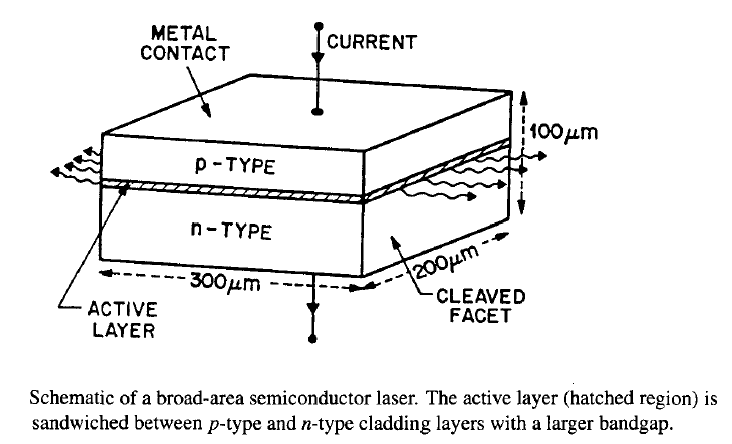The central core layer is made of the semiconductor that emits light and is called the "active" layer. The cladding layers are made using a semiconductor whose bandgap is larger than that of the active layer. The bandgap difference between the two semiconductors helps to confine the electrons and holes to the active layer. At the same time, the active layer has a slightly larger refractive index than the surrounding cladding layers that acts a planar waveguide whose number of modes can be controlled by changing the active-layer thickness. The main point is that such a heterostructure design helps to confine both the injected carriers (electrons and holes) and the light generated within the active layer through electron-hole recombination. A third feature is that the two cladding layers are transparent to the emitted light by virtue of their higher bandgap, thereby resulting in a low-loss structure. These features have made semiconductor lasers practical for a wide variety of applications.

When the injected carrier density in the active layer exceeds a certain value, population inversion is realized and the active region exhibits optical gain. An input signal propagating inside the active layer is then amplified by a factor of exp(gL), where g is the gain coefficient and L is the active-layer length. The calculation of g requires the rates at which photons are absorbed and emitted through stimulated emission and depends on details of the band structure associated with the active material. In general, g is calculated numerically. The following figure (a) shows the gain calculated for a 1.3-μm InGaAsP active layer at different values of the injected carrier density N. For N =  1 x 1018 cm-3, g < 0, as population inversion has not yet occurred. As N increases, g becomes positive over a spectral range that increases with N. The peak value of the gain, gp, also increases with N, together with a shift of the peak toward higher photon energies. The variation of gp with N is shown in (b). For N > 1.5 x1018 cm-3, gp varies almost linearly with N. This figure shows that the optical gain in semiconductors increases rapidly once population inversion is realized. It is because of such a high gain that semiconductor lasers can be made with physical dimensions of less than 1 mm.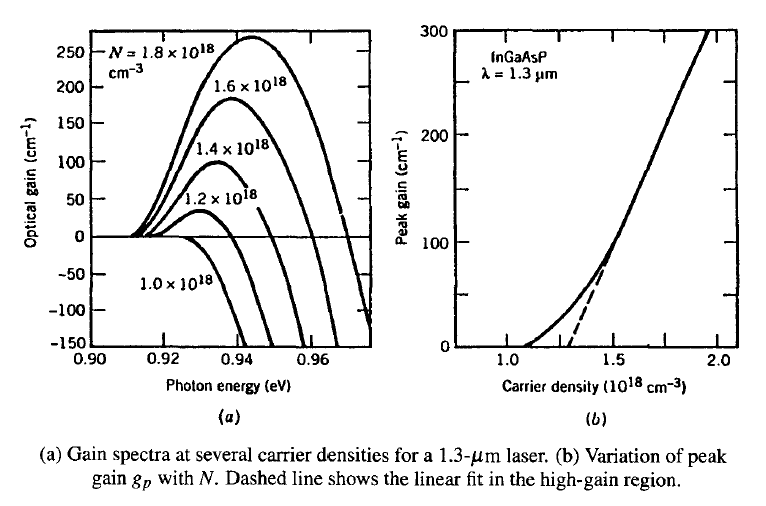The nearly linear dependence of gp on N suggests an empirical approach in which the peak gain is approximated by

gp(N) = σg(N - NT)

where NT is the transparency value of the carrier density and σg is the gain cross section;  σg is also called the differential gain. Typical values of NT and σg for InGaAsP lasers are in the range 1.0-1.5 x 1018 cm-3 and 2-3 x 10-16 cm-2, respectively. As seen the (b) figure above, this approximation is reasonable in the high-gain region where gp exceeds 100 cm-1; most semiconductor lasers operate in this region. The use of this approximation simplifies the analysis considerably, as band-structure details do not appear directly. The parameters σg and NT can be estimated from numerical calculations such as those shown in (b) figure above or can be measured experimentally.

Semiconductor lasers with a larger value of σg generally perform better, since the same amount of gain can be realized at a lower carrier density or, equivalently, at a lower injected current. In quantum-well semiconductor lasers, σg is typically larger by about a factor of 2. The linear approximation in the equation above for the peak gain can still be used in a limited range. A better approximation replaces it with gp(N) = g0[1 + ln(N/N0)], where gp =  g0 at N = N0 and N0 = eNT ≈ 2.718NT by using the definition gp = 0 at N = NT.

#### 4. Feedback and Laser Threshold

The optical gain alone is not enough for laser operation. The other necessary ingredient is optical feedback, which converts any amplifier into an oscillator. In most lasers the feedback is provided by placing the gain medium inside a Fabry-Perot (FP) cavity formed by using two mirrors. Semiconductor lasers do not require external mirrors because the two cleaved facets can act as mirrors because of a relatively large index difference across the air-semiconductor interface. The facet reflectivity normal to this interface is given by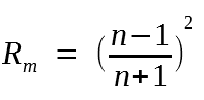where n is the refractive index of the gain medium. Typically, n = 3.5, resulting in 30% facet reflectivity. Even though FP cavity formed by two cleaved facets is relatively lossy, the gain in a semiconductor laser is large enough that high losses can be tolerated.

A simple way to obtain the threshold condition is to study how the amplitude of an optical mode changes during one round trip inside the FP cavity. Assume that the mode has initially an amplitude A0, frequency ν, and propagation constant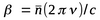, where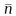is the mode index. After one round trip, its amplitude increases by exp[2(g/2)L] because of gain (g is the power gain) and its phase changes by 2βL, where L is the length of the laser cavity. At the same time, its amplitude decreases by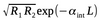because of reflection at the laser facets and because of internal losses αint resulting from free-carrier absorption and interface scattering. The facet reflectivities R1 and R2 can be different if facets are coated to change their natural reflectivity. In the steady state, the mode should remain unchanged after one round trip, that is: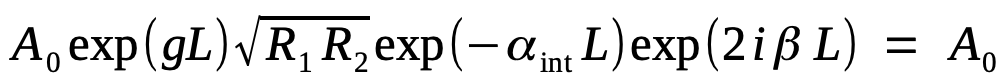By equating the amplitude and the phase on two sides, we obtain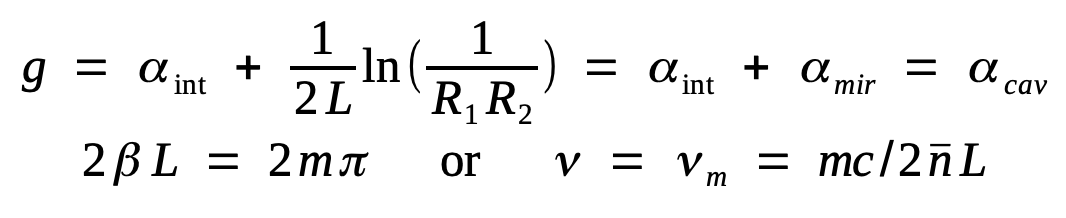where m is an integer. The first equation shows that the gain g equals total cavity loss αcav at the threshold and beyond. It is important to note that g is not the same as the material gain gm shown in the previous figure. The optical mode extends beyond the active layer while the gain exists only inside it. As a result, g = Γgm, where Γ is the confinement factor of the active region with typical values < 0.4.

#### 5. Longitudinal Modes

The phase condition in the above equation shows that the laser frequency ν must match one of the frequencies in the set νm, where m is an integer. These frequencies correspond to the longitudinal modes and are determined by the optical length. The spacing ΔνL between the longitudinal modes is constant. In fact, it is the same as the free spectral range associated with any FP resonator and is given by ΔνL = c/2ngL when material dispersion is included, where ng is the group index. Typically, ΔνL = 150 GHz for L = 250 μm.

A semiconductor laser generally emits light in several longitudinal modes of the cavity simultaneously.As seen in the figure above, the gain spectrum g(ω) of semiconductor lasers is wide enough (bandwidth ~10 THz) that many longitudinal modes of the FP cavity experience gain simultaneously. The mode closest to the gain peak becomes the dominant mode. Under ideal conditions, the other modes should not reach threshold since their gain always remains less than that of the main mode. In practice, the difference is extremely small (~0.1 cm-1) and one to two neighboring modes on each side of the main mode carry a significant portion of the laser power together with the main mode.

Since each mode propagates inside the fiber at a slightly different speed because of group-velocity dispersion, the multimode nature of a semiconductor laser often limits the bit rate of lightwave systems operating near 1.55 μm. Performance can by improved by designing lasers such that they oscillate in a single longitudinal mode. We will discuss that in the next tutorial.

#### 6. Laser Structures

The simplest structure of a semiconductor laser consists of a thin active layer (with a thickness of 0.1 μm or less) sandwiched between the p-type and n-type cladding layers of another semiconductor with a higher bandgap. Such lasers are called broad-area lasers since the current is injected over a relatively broad area covering the entire width of the laser chip. The laser light is emitted from the two cleaved facets in the form of an elliptic spot of dimension ~1 x 100 μm2.  In the transverse direction perpendicular to the junction plane, the spot size is ~1 μm because the active layer supports only the fundamental TE0 and TM0 modes. In practice, gain is slightly larger for the TE0 mode, and the laser light is polarized in the junction plane. Since no confinement mechanism exists in the lateral direction (parallel to the junction plane), emitted light spreads over the entire width of a broad-area laser, resulting in a highly elliptical beam. Such lasers suffer from a number of deficiencies and are rarely used in practice. The major drawbacks are a relatively high threshold current and a spatial pattern that changes in an uncontrollable manner with the current. These problems can be solved by introducing a mechanism for light confinement in the lateral direction.

The light-confinement problem is solved in the so-called index-guided semiconductor lasers by introducing an index step ΔnL in the lateral direction so that a rectangular waveguide is formed. The following figure shows two commonly used designs.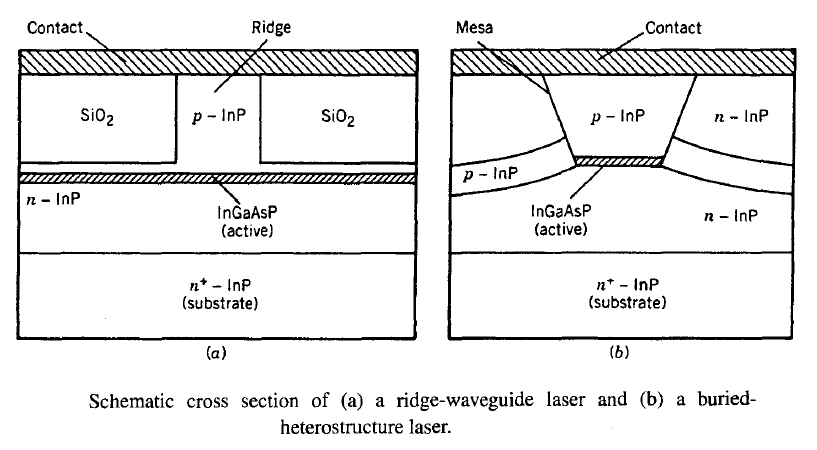In a ridge-waveguide laser (a), a ridge is formed by etching most of the top cladding layers. A silica layer is then deposited to block the current flow so that the current enters only through the ridge. Since the cladding material used for the ridge has a much larger refractive index than silica, the mode index is also higher under the ridge, resulting in an index step ΔnL ≈ 0.01. This index difference guides the optical mode in the lateral direction. The magnitude of the index step is sensitive to many fabrication details, such as the ridge width and the proximity of the silica layers to the active layer. Although this scheme offers only weak lateral confinement, the relative simplicity of the ridge-waveguide design and the resulting low cost make this design attractive for some applications.

In strongly index-guided semiconductor lasers, the active region is buried on all sides by several layers of lower refractive index (typical dimensions ~0.1 x 1 μm2). Such lasers are called buried heterostructure (BH) lasers (see figure b). Several different kinds of BH lasers have been developed. They are known under names such as etched-mesa BH, planar BH, double-channel planar BH, and V-grooved or channelled substrate BH lasers, depending on the fabrication method used to realize the laser structure. They all allow a relatively large index step ( ΔnL > 0.1) in the lateral direction and, as a result, permit strong mode confinement. Because of a large built-in index step, the spatial distribution of the emitted light is inherently stable, provided that the laser is designed to support a single spatial mode. In practice, a BH laser operates in a single mode if the active-region width is reduced to below 2 μm. The spot size still remains elliptical with typical dimensions of 2 x 1 μm2. Because of  small spot-size dimensions, the output beam diffracts considerably in both the lateral and transverse directions as it leaves the laser. An elliptic spot size and a large divergence angle make it difficult to couple light into the fiber efficiently. A spot-size converter is sometimes used to improve the coupling efficiency.